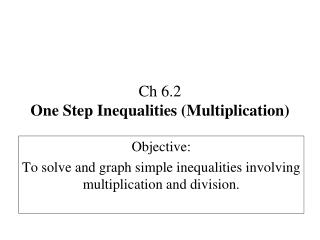DownloadDownload PresentationCh 6.2 One Step Inequalities (Multiplication)

# Ch 6.2 One Step Inequalities (Multiplication)

Download Presentation## Ch 6.2 One Step Inequalities (Multiplication)

- - - - - - - - - - - - - - - - - - - - - - - - - - - E N D - - - - - - - - - - - - - - - - - - - - - - - - - - -
##### Presentation Transcript

1. Ch 6.2One Step Inequalities (Multiplication) Objective: To solve and graph simple inequalities involving multiplication and division.

2. Definitions • Multiplication Property of Inequality: When multiplying by a negative number, the inequality symbol reverses. In other words, “FLIP” the inequality sign • Division Property of Inequality: When dividing by a negative number, the inequality symbol reverses. In other words, “FLIP” the inequality sign

3. Rules Inequalities transform like equations except... When multiplying or dividing by a negative number you must reverse (flip) the inequality. -4 -3 -2 -1 0 1 2 3 4 Negative side Positive side -3 < -2 3 > 2 (-1)(-1) 3 > 2 Large is small Large is large flip -3 -2 <

4. Why? Example using Multiplication/Division: -x < 1 -1 -1 x > -1 (The inequality “flipped”) Same example using Addition/Subtraction: - x < 1 + x + x 0 < 1 + x - 1 < -1 -1 < x = x > -1

5. Example 1 Example 2 (3) (3) x -6 x 5 ≤ > -6 0 0 5 Example 4 Example 3 (-3) (-3) y -21 ≤ x -6 > -21 0 -6 0

6. Example 6 Example 5 (-2) (-2) x -6 < m -12 > -12 0 -6 0 Example 8 Example 7 (8) (8) -13 n n > -13 < x -2 ≥ -13 0 -2 0

7. Classwork Graph the following inequalities. 1) 2) 0 0 3) 4) 0 0

8. 5) 6) 0 0 7) 8) -2k < 8 0 0